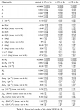Plots for New Physics in B-B mixing
in the light of recent LHCb data

Reference:

 Some of the plots below come from the following paper: New Physics in B-B mixing in the light of recent LHCb data by A. Lenz et al., whereas the others are additional plots obtained with the same inputs. This update includes the results up to the end of 2011, including the CDF [arXiv:1112.1726] and LHCb [arXiv:1112.3183] results on (φs,ΔΓs) from Bs->J/Ψ Φ. It corresponds to the second version of arXiv:1203.0238 (the first version is available here).

Numerical results:

 The results of the update include: Inputs of the fit Values of the CKM and NP parameters, Predictions of selected observables, for the model-independent scenario I of New Physics (NP) in ΔF=2Numerical Results

Scenario I:

In scenario I we have introduced NP in M12q = M12SM,qΔq independently for Bd, Bs and K, corresponding to NP with arbitrary flavour structure.

Constraint for Bd mixing:
Re Δd=0.823+0.143-0.095, and Im Δd=-0.199+0.062-0.048.eps png
Constraint for Bs mixing:
Re Δs=0.965+0.133-0.078,
and Im Δs=0.00+0.10-0.10.

The pulls for the relevant observables are 0.7 σ for B->τν, 2.7 σ for φsJ/Ψ Φ and 2.9 σ for ASL.eps png
Indirect constraint on the asymmetry ASL=(-15.6+9.2-3.9)10-4 compared with the direct measurements of CDF and D0.eps png
Indirect constraint on the CP phase φsJ/Ψ Φ=-56.8+10.9-7.0ˆ compared with the direct measurements of CDF, D0 and LHCb.eps png

Scenario II:

In scenario II we have introduced NP in M12q = M12SM,qΔq with Minimal Flavour Violation (MFV) with small bottom Yukawa coupling yielding Δds=Δ real.

Constraint for neutral meson mixing Δ=0.920+.120-0.039.eps png

Scenario III:

In scenario III we have introduced NP in M12q = M12SM,qΔq with Minimal Flavour Violation (MFV) with large bottom Yukawa coupling yielding Δds=Δ complex.

Constraint for Bd and Bs mixings:
Re Δ=0.90+0.29-0.11, and Im Δ=-0.171+0.061-0.052.eps png

Scenario IV:

In order to accomodate the measurements of φs and ASL, we introduce a fourth scenario with NP occurring not only in M12q, but also Gamma;12q. We introduce the new parameters

δq = (Γ12q/M12q)/(Re[Γ12SM,q/M12SM,q)

with real and imaginary parts related to ΔΓq/ΔMq and aSLq. Re[δd] is weakly constrained experimentally, contrary to the three other quantities. The best for the SM predictions are δsSM = 1 − 0.0059 i and δdSM = 1. + 0.097 i.
Constraint for Im(δd) and Im(δs) with the 68% CL intervals Im(δd)=0.92+1.13-0.69 and Im(δs)=1.2+1.6-1.0. The p-value for the 2D SM hypothesis Im(δd)= Im(δdSM) and Im(δs)= Im(δsSM) is 3.2 σ.eps png
Constraint for Re(δs) and Im(δs). The p-value for the 8D SM hypothesis δd,s= δd,sSM and Δds=1 is 2.6 σ.eps png